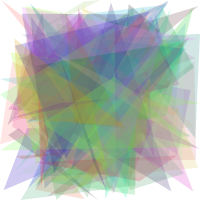Suppose we want to draw a batch of images, where each image is made up of randomly positioned and colored triangles, that are blending. It will look like this:and then find the euclidean distance of each such image to a given target image.

Now why on earth am I doing this? Well, this turns into an interesting optimization problem of finding the closest triangle image and also an excuse to practise Julia. The inspiration is from the this repo based on EvoJax.

Towards framing this as an optimization problem, we will represent a triangle as a vector of size 10, made up of floating point numbers between 0 and 1. Four numbers of this vector are for the color of the triangle; r,g,b and alpha, and for the three vertices of the triangle we need 6 numbers, (x1,y1), (x2,y2), (x3,y3). Hence, if we want to draw M images, each image having N triangles, we need a matrix of size (10,N,M), which will be our parameters matrix. I want to randomly create such a matrix and min-max scale it along the triangle dimension, by which I mean, for each image, I first find the minimum and maximum of a triangle parameter among the N triangles, and then subtract from the parameter this minimum and then divide the result by the difference between the maximum and the minimum. I want to end up with an array of size (3,w,h,M) for the images, where w is width and h is height, and an array of size M for the distances. Let’s see how fast we can do this.

First order of business is setting this up, note that I am scaling the numbers to the given width and height:

## With a 2d library

The first thing that comes to mind is to use a 2d graphics library for drawing, and since the Cairo lib is available, let’s try that. The function below is drawing the triangles on a blank white Cairo canvas, and copying it to the img array at the end:

Now let’s draw each image in this fashion:

Benchmarking this with `@btime withCairo();`

I see `428.101 ms (3157 allocations: 205.09 MiB)`.

## The cross product method

Now the cool part. Move your mouse inside and outside of the triangle below. You will see a bar chart, depicting the magnitude and direction of the 3rd components of the cross products, AB with AP (reds), BC with BP (greens) and CA with CP (blues). Observe that all those bars point to the same direction ONLY inside the triangle!

Whats great about this is that, cross products are just multiplications and subtractions, perfect job to parallelize with a GPU.

So, what needs to be done is clear. For each of the M images, and for each of the N triangles, our operation is to update a pixel color to blend with the current triangle’s color, if that pixel is inside the triangle. We will parallelize this operation with a CUDA kernel, shown below:

We will need to pass our parameters and target array to the GPU, and then call the kernel with `@cuda`. We can create white canvases with `CUDA.ones` here, so no need to pass it.

Benchmarking this I see `120.315 ms (38689 allocations: 52.53 MiB)`

That’s about 4x speedup, not really impressive, but perhaps not too bad considering I have an old GPU. Note that I benchmarked with `--check-bounds=no`, which is a startup option that you pass to Julia, when launching, that disables the performance killer “bounds checking”.

In the next post, I will talk about the very cool and general PGPE algorithm used in EvoJax to steer these images towards a target image. You can see one example of this here.